Home
Hostname: page-component-59b7f5684b-9m8n8 Total loading time: 0.464 Render date: 2022-10-03T03:07:28.692Z Has data issue: true Feature Flags: { "shouldUseShareProductTool": true, "shouldUseHypothesis": true, "isUnsiloEnabled": true, "useRatesEcommerce": false, "displayNetworkTab": true, "displayNetworkMapGraph": true, "useSa": true } hasContentIssue true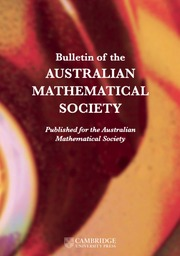Bulletin of the Australian Mathematical Society

## Abstract

A subset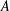$A$ of a finite abelian group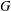$G$ is called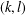$(k,l)$-sum-free if the sum of$k$ (not necessarily distinct) elements of$A$ never equals the sum of$l$ (not necessarily distinct) elements of$A$. We find an explicit formula for the maximum size of a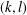$(k,l)$-sum-free subset in$G$ for all$k$ and$l$ in the case when$G$ is cyclic by proving that it suffices to consider$(k,l)$-sum-free intervals in subgroups of$G$. This simplifies and extends earlier results by Hamidoune and Plagne [‘A new critical pair theorem applied to sum-free sets in abelian groups’, Comment. Math. Helv. 79(1) (2004), 183–207] and Bajnok [‘On the maximum size of a$(k,l)$-sum-free subset of an abelian group’, Int. J. Number Theory 5(6) (2009), 953–971].

## MSC classification

Type
Research Article
Information
© 2018 Australian Mathematical Publishing Association Inc.

## Access options

Get access to the full version of this content by using one of the access options below. (Log in options will check for institutional or personal access. Content may require purchase if you do not have access.)

## References

Bajnok, B., ‘On the maximum size of a (k, l)-sum-free subset of an abelian group’, Int. J. Number Theory 5(6) (2009), 953971.CrossRefGoogle Scholar
Bajnok, B., Additive Combinatorics: A Menu of Research Problems (CRC Press, Boca Raton, FL, 2018).CrossRefGoogle Scholar
Balasubramanian, R., Prakash, G. and Ramana, D. S., ‘Sum-free subsets of finite abelian groups of type III’, European J. Combin. 58 (2016), 181202.CrossRefGoogle Scholar
Bier, T. and Chin, A. Y. M., ‘On (k, l)-sets in cyclic groups of odd prime order’, Bull. Aust. Math. Soc. 63(1) (2001), 115121.CrossRefGoogle Scholar
Chervak, O., Pikhurko, O. and Staden, K., ‘Minimum number of additive tuples in groups of prime order’, Preprint, 2017, arXiv:1710.01936 [math.CO].Google Scholar
Diananda, P. H. and Yap, H. P., ‘Maximal sum-free sets of elements of finite groups’, Proc. Japan Acad. 45 (1969), 15.CrossRefGoogle Scholar
Erdős, P., ‘Extremal problems in number theory’, in: Proc. Sympos. Pure Mathematics, Vol. VIII (American Mathematical Society, Providence, RI, 1965), 181189.Google Scholar
Green, B. and Ruzsa, I., ‘Sum-free sets in abelian groups’, Israel J. Math. 147 (2005), 157188.CrossRefGoogle Scholar
Hamidoune, Y. O. and Plagne, A., ‘A new critical pair theorem applied to sum-free sets in abelian groups’, Comment. Math. Helv. 79(1) (2004), 183207.CrossRefGoogle Scholar
Plagne, A., ‘Maximal (k, l)-free sets in ℤ/pℤ are arithmetic progressions’, Bull. Aust. Math. Soc. 65(3) (2002), 137144.CrossRefGoogle Scholar
Samotij, W. and Sudakov, B., ‘The number of additive triples in subsets of abelian groups’, Math. Proc. Cambridge Philos. Soc. 160(3) (2016), 495512.CrossRefGoogle Scholar
Samotij, W. and Sudakov, B., ‘The number of additive triples in subsets of abelian groups’, Preprint, 2015, arXiv:1507.03764 [math.NT].Google Scholar
Street, A. P., ‘Maximal sum-free sets in abelian groups of order divisible by three’, Bull. Aust. Math. Soc. 6 (1972), 439441.CrossRefGoogle Scholar
Street, A. P., ‘Corrigendum: “Maximal sum-free sets in abelian groups of order divisible by three”’, Bull. Aust. Math. Soc. 7 (1972), 317318.CrossRefGoogle Scholar
Wallis, W. D., Street, A. P. and Wallis, J. S., Combinatorics: Room Squares, Sum-free Sets, Hadamard Matrices, Lecture Notes in Mathematics, 292 (Springer, Berlin–New York, 1972).CrossRefGoogle Scholar
Yap, H. P., ‘Maximal sum-free sets in finite abelian groups. II’, Bull. Aust. Math. Soc. 5 (1971), 4354.CrossRefGoogle Scholar
1
Cited by

# Save article to Kindle

To save this article to your Kindle, first ensure coreplatform@cambridge.org is added to your Approved Personal Document E-mail List under your Personal Document Settings on the Manage Your Content and Devices page of your Amazon account. Then enter the ‘name’ part of your Kindle email address below. Find out more about saving to your Kindle.

Note you can select to save to either the @free.kindle.com or @kindle.com variations. ‘@free.kindle.com’ emails are free but can only be saved to your device when it is connected to wi-fi. ‘@kindle.com’ emails can be delivered even when you are not connected to wi-fi, but note that service fees apply.

Find out more about the Kindle Personal Document Service.

THE MAXIMUM SIZE OF$(k,l)$-SUM-FREE SETS IN CYCLIC GROUPS Available formats × # Save article to Dropbox To save this article to your Dropbox account, please select one or more formats and confirm that you agree to abide by our usage policies. If this is the first time you used this feature, you will be asked to authorise Cambridge Core to connect with your Dropbox account. Find out more about saving content to Dropbox. THE MAXIMUM SIZE OF$(k,l)$-SUM-FREE SETS IN CYCLIC GROUPS
Available formats
×

# Save article to Google Drive

To save this article to your Google Drive account, please select one or more formats and confirm that you agree to abide by our usage policies. If this is the first time you used this feature, you will be asked to authorise Cambridge Core to connect with your Google Drive account. Find out more about saving content to Google Drive.

THE MAXIMUM SIZE OF$(k,l)\$-SUM-FREE SETS IN CYCLIC GROUPS
Available formats
×
×